## LetsPlayMaths.Com

WELCOME TO THE WORLD OF MATHEMATICS

# Class 3 Capacity / Volume

Capacity / Volume

Conversion of Liters into Milliliters

Conversion of Milliliters into Liters

Addition of Liters and Milliliters

Subtraction of Liters and Milliliters

Multiplication of Liters and Milliliters

Division of Liters and Milliliters

Capacity / Volume Test

Capacity / Volume Worksheet

Answer Sheet

## Capacity / Volume

Capacity is measured in liters and milliliters. Or, we can say standard unit of capacity is one liter. One liter is further subdivided into 1000 equal parts, each small part is called 1 milliliter. Water, milk, fuel, etc. are measured in liters and liquid medicines are measured in milliliters.

So, 1 liter = 1000 milliliters
Short form of 1 liter is l. We write 5 liters as 5 l.
Short form of milliliter is ml. So, 10 milliliters can be written as 10 ml.

## Conversion of Liters into Milliliters

To convert liters into milliliters, we have to multiply 1000 with the number of liters.

Example 1. Convert 6 liters into milliliters

Solution. 6 liters = 6 X 1000

Example 2. Convert 15 l 750 ml into milliliters.

Solution. 15 l 750 ml = 15 liters + 750 ml = 15 X 1000 ml + 750 ml
= 15000 ml + 750 ml = 15750 ml

## Conversion of Milliliters into Liters

Last three digits from right give the milliliter and remaining digits on the left give the liters.

Example 1. Convert 7000 milliliters into liters.

Solution. 7 000 ml = 7 l 0 ml
So, 7000 ml is equal to 7 l.

Example 2. Convert 26579 ml into liter and milliliter.

Solution. 26579 ml = 26000 ml + 579 ml = 26 X 1000 ml + 579 ml = 26 l 579 ml.
Other way 26 579 ml = 26 l 579 ml

## Addition of Liters and Milliliters

Addition of capacity can be done in two methods, they are given below with examples.

Method 1.
Step 1. Convert the milliliters into three-digit format, for example, 5 l 8 ml should be written as 5 l 008 ml.

Step 2. Add milliliters first. If the result is of 3 digits, then it should be written as milliliters. If the result is

of 4 digits, then the left most digit should be carry over to liters column.

Step 3. Add the liters.

Example 1. Add 123 l 57 ml and 45 l 245 ml

Solution. Write the 123 l 57 ml and 45 l 245 ml in column format as shown below.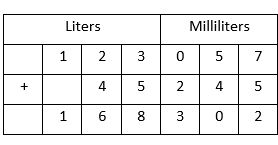Step 1. 123 l 57 ml is written as 123 l 057 ml in the table.

Step 2. Add the milliliters

245 ml + 057 ml = 302 ml

Write 302 ml under milliliters column

Step 3. Add liters, 123 l + 45 l = 168 ml. Write 168 l under liters column.

So, the answer is 168 l 302 ml.

Method 2.
We can add liters and milliliters like normal numbers, but milliliters should be written as three digits numbers. For example, 45 milliliters should be written as 045 ml and 5 milliliters should be written as 005 ml.

Example 1. Add 452 l 125 ml, 126 l 457 ml and 8 l 5 ml.

Solution. Write 452 l 125 ml, 126 l 457 ml and 8 l 5 ml in tabular format.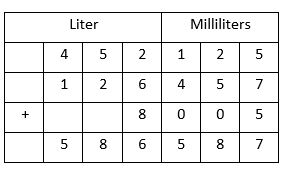So, the answer is 586 l 587 ml

## Subtraction of Liters and Milliliters

This kind of subtraction can be done in two methods, they are given below with examples.

Method 1.
Step 1. Subtract the milliliters first. If we had to borrow 1 from liter, then it must be converted to milliliters
first that is 1000 ml and then perform the subtraction.
Step 2. Subtract the liters. If we borrowed 1 l for milliliters subtraction, then we must reduce 1 l from the liters.

Example 1. Subtract 78 l 750 ml from 197 l 250 ml

Solution. Arrange 197 l 250 ml and 78 l 750 ml in tabular format.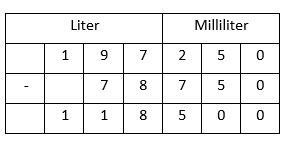Step 1. Subtract milliliters. 750 ml > 250 ml, so we have to borrow 1 l from 197 l.

1 l + 250 ml = 1000 ml + 250 ml = 1250 ml
1250 ml – 750 ml = 500 ml, Write 500 ml under milliliters column.
Step 2. Since we borrowed 1 l, so 197 l becomes 196 l. Now subtract 78 l from 196 l.

196 l – 78 l = 118 l. Write 118 l under liters column.
So, the answer is 118 l 500 ml.

Method 2.
We can subtract liters and milliliters as normal subtraction. But, milliliters should be written as three-digit numbers.

Example 1. Subtract 426 l 546 ml from 526 l 126 ml.

Solution. Write 426 l 546 ml and 526 l 126 ml in tabular format.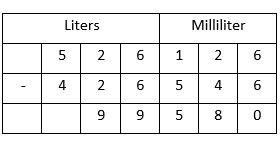So, the answer is 99 l 580 ml.

## Multiplication of Liters and Milliliters

Multiplication of liters and milliliters can be done in two methods.

Method 1.
Step 1. Multiply milliliters first. If the result is consisting of three digits, then write it under milliliters column.

But, if the result is of four digits then carry the 1st digit from left to liters columns for addition.

Step 2. Multiply liters, add the result with carryover from the milliliters column.

Example 1. Multiply 82 l 135 ml by 6.

Solution. Write the numbers in tabular format as show below.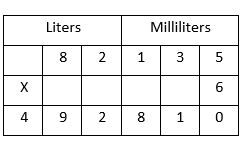Step 1. Multiply grams first. 135 ml X 6 = 810 ml

Write 810 ml under milliliters column.
Step 2. Multiply liters next. 82 l X 6 = 492 l
Write 492 l under liter column.
So, the answer is 492 l 810 ml.

Method 2.
We can multiply liters and milliliters as normal multiplication. But, milliliters should be written as three-digit numbers.

Example 1. Multiply 326 l 9 ml by 4
Solution. Write 326 l 9 ml and 4 in tabular format as shown below.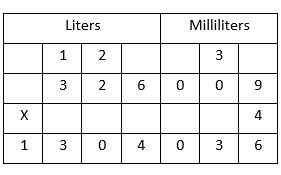So, the answer is 1304 l 36 ml.

## Division of Liters and Milliliters

Division of liters and milliliters can be done in two methods.

Method 1.
Step 1. Convert liters and milliliters into milliliters.
Step 2. Divide the milliliters with divisor like normal division.
Step 3. Now convert the quotient to liters and milliliters.

Example 1. Divide 94 l 464 ml by 4
Solution. 94 l 464 ml is equals to 94464 ml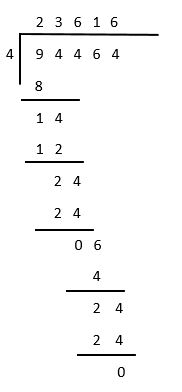So, the answer is 23 l 616 ml.

Method 2.
Division of liters and milliliters can be done with normal division. In this method, grams must be written in 3-digit format.

Example 1. Divide 26 l 525 ml by 5
Solution.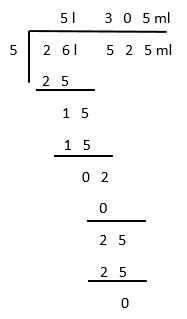So, the answer is 5 l 305 ml.

## Capacity / Volume Test

Capacity Test - 1

## Capacity / Volume Worksheet

Capacity Worksheet - 1

Capacity Worksheet - 2

## Answer Sheet

Capacity-AnswerDownload the pdf

Copyright © 2021 LetsPlayMaths.com. All Rights Reserved.